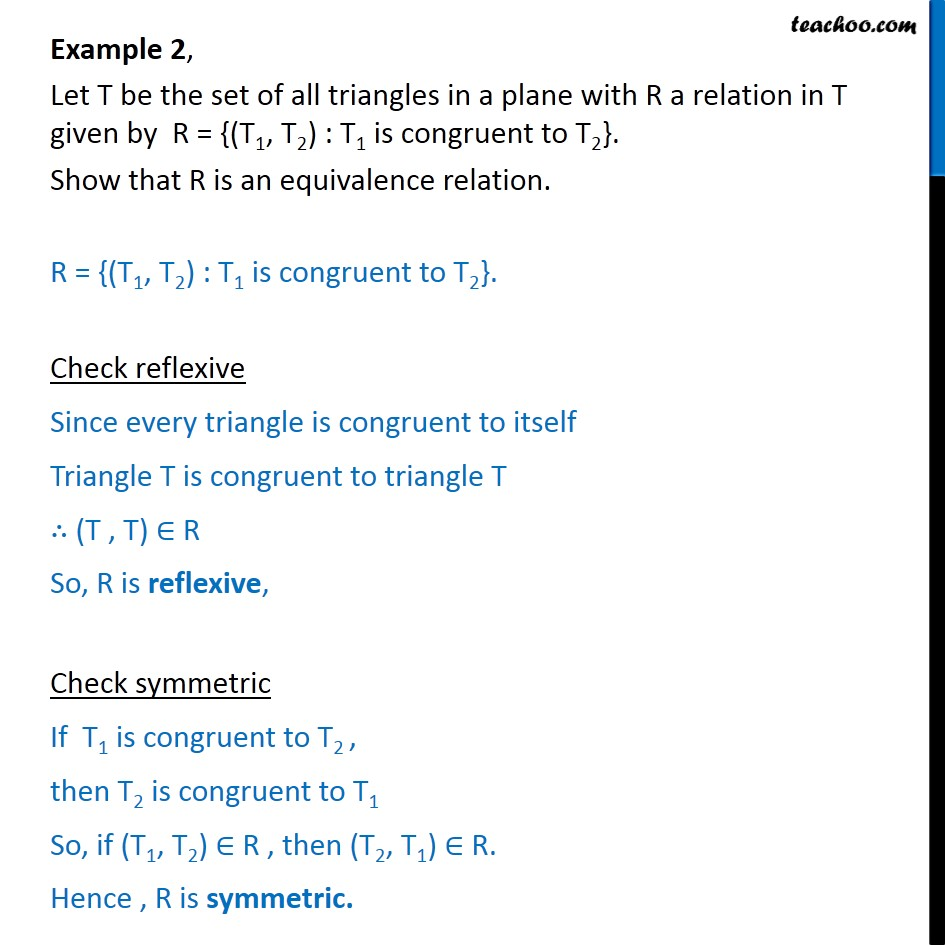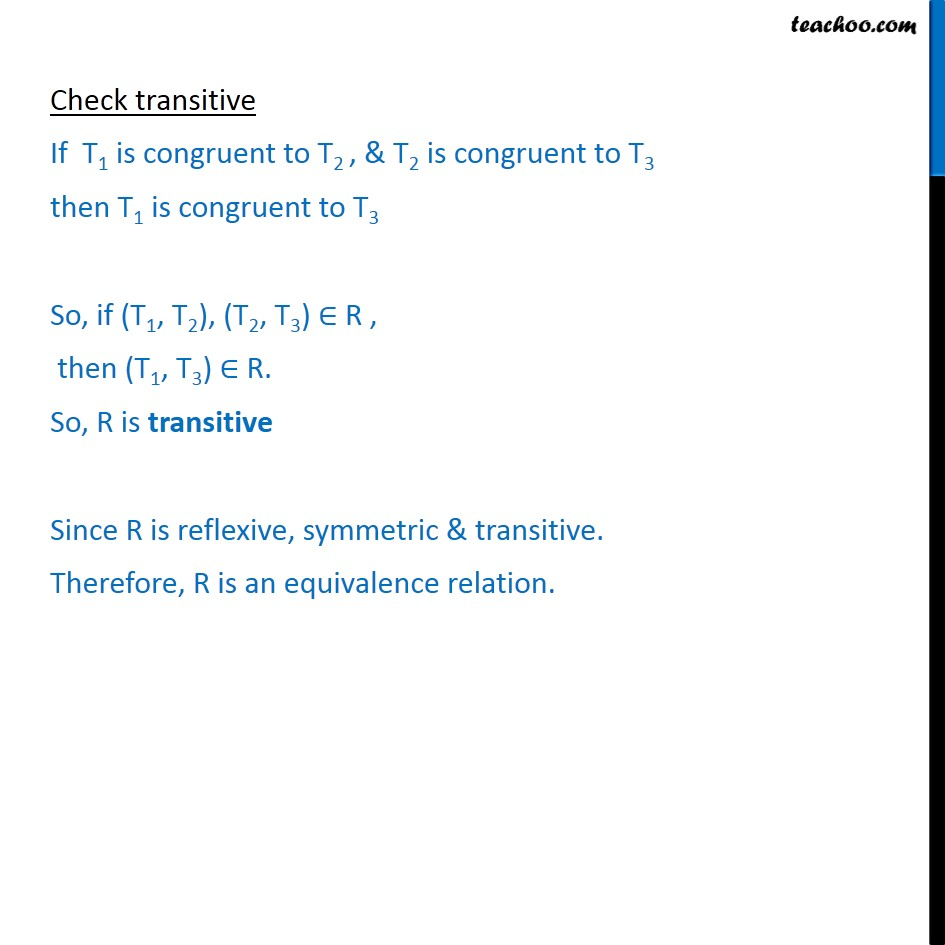To prove relation reflexive, transitive, symmetric and equivalent

Chapter 1 Class 12 Relation and Functions
Concept wiseIntroducing your new favourite teacher - Teachoo Black, at only ₹83 per month

### Transcript

Example 2, Let T be the set of all triangles in a plane with R a relation in T given by R = {(T1, T2) : T1 is congruent to T2}. Show that R is an equivalence relation. R = {(T1, T2) : T1 is congruent to T2}. Check reflexive Since every triangle is congruent to itself Triangle T is congruent to triangle T ∴ (T , T) ∈ R So, R is reflexive, Check symmetric If T1 is congruent to T2 , then T2 is congruent to T1 So, if (T1, T2) ∈ R , then (T2, T1) ∈ R. Hence , R is symmetric. Check transitive If T1 is congruent to T2 , & T2 is congruent to T3 then T1 is congruent to T3 So, if (T1, T2), (T2, T3) ∈ R , then (T1, T3) ∈ R. So, R is transitive Since R is reflexive, symmetric & transitive. Therefore, R is an equivalence relation.